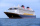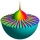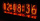# Boats

Three-quarters of boats are white, 1/7 are blue and 9 are red. How many boats do we have?

n =  84

### Step-by-step explanation:

3/4n + 1/7n + 9 = n

3/4•n + 1/7•n + 9 = n

3n = 252

n = 84

Our simple equation calculator calculates it.Did you find an error or inaccuracy? Feel free to write us. Thank you!

Showing 1 comment:Dr Math
An example in original writing: "three-quarters of boats are white, 4/7 are blue and 9 are red. How many boats do we have? " have no solution because of 3/4 + 4/7 = 37/28 > 1

And equation: 3/4n + 4/7n + 9 = n has negative solution n=-28

https://www.hackmath.net/en/calculator/fraction?input=3%2F4+%2B+4%2F7&submit=CalculateTips to related online calculators
Need help to calculate sum, simplify or multiply fractions? Try our fraction calculator.
Do you have a linear equation or system of equations and looking for its solution? Or do you have a quadratic equation?

## Related math problems and questions:

• Simplify 3Simplify mixed numerals expression: 8 1/4- 3 2/5 - (2 1/3 - 1/4) Show your solution.
• Sum of fractionsWhat is the sum of 2/3+3/5?
• Mixed numbersRewrite mixed numbers, so the fractions have the same denominator: 5 1/5 - 2 2/3
• Turtles 2A box turtle hibernates in the sand at 11 5/8. A spotted turtle hibernates at 11 16/25 feet. Which turtle is deeper? Write answer as number 1 or 2.
• Here areHere are white, blue, and red boats in a marina. Two-thirds of the boats in the marina are white, 4/7 of the remaining boats are blue, and the rest are red. If there are 15 red boats, how many boats are in the marina? Which tape diagram represents how man
• Eq2 2Solve following equation with quadratic members and rational function: (x2+1)/(x-4) + (x2-1)/(x+3) = 23
• Fraction + eqSolve following simple equation with fractions: -5/6(8+5b) = 75 + 5/3b
• Boats 2Three quarters of the boats in the marina are white 4/7 of the remaining boats are blue, rest are red if there are 9 red boats. How many boats are in the marina?
• ComplaintsThe table is given: days complaints 0-4 2 5-9 4 10-14 8 15-19 6 20-24 4 25-29 3 30-34 3 1.1 What percentage of complaints were resolved within 2weeks? 1.2 calculate the mean number of days to resolve these complaints. 1.3 calculate the modal number of day
• Evaluate 5Evaluate expression x2−7x+12x−4 when x=−1
• LCD two fractionsWhat is the least common denominator of 11/15 and 12/19?
• Linear combination of complexIf z1=5+3i and z2=4-2i, write the following in the form a+bi a) 4z1+6z2 b) z1*z2
• Solve 2Solve integer equation: a +b+c =30 a, b, c = can be odd natural number from this set (1,3,5,7,9,11,13,15)
• Box and whisker plotConstruct a box and whisker plot for the given data. 56, 32, 54, 32, 23, 67, 23, 45, 12, 32, 34, 24, 36, 47, 19, 43
• EmilyEmily had 20 minutes to do a three-problem quiz. She spent 11 3/4 minutes on question A and 5 1/2 minutes on question B. How much time did she have left for question C?
• Standard deviationFind standard deviation for dataset (grouped data): Age (years) No. Of Persons 0-10 15 10-20 15 20-30 23 30-40 22 40-50 25 50-60 10 60-70 5 70-80 10
• Prime factorsWrite 98 as a product of prime factors